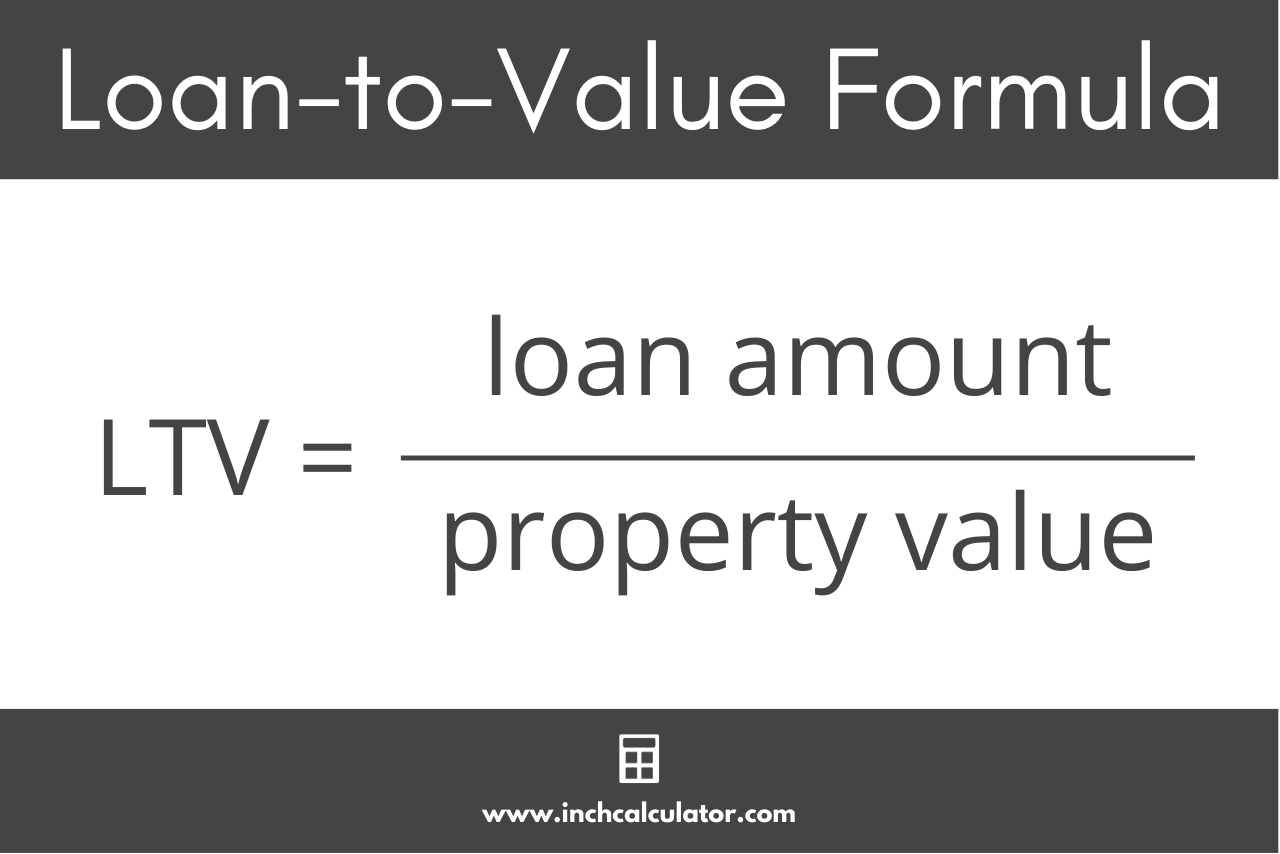# LTV Calculator – Calculate Loan-to-Value Ratio

Use our LTV calculator to calculate a loan-to-value ratio for a home purchase or refinance.

\$
\$
\$
\$
\$

## Loan-to-Value:

### Loan Amount

\$
Learn how we calculated this below

## How to Calculate a Loan-to-Value Ratio

When you are applying for a loan, one of the most important things that lenders will look at is the loan-to-value (LTV) ratio. LTV is a ratio of the amount of money you want to borrow compared to the value of the property that you are purchasing or own and will be used as collateral for the loan.

Lenders use this ratio in addition to your debt-to-income ratio to assess the level of risk they are taking when lending.

The higher the LTV ratio, the more risk the lender is taking on because there is less collateral in property value if you default on your loan.

A lower LTV ratio indicates that you have more equity, and thus more collateral, in the event that you default on the loan.

### Loan-to-Value Formula

You can use the loan-to-value formula to calculate the LTV ratio:

LTV = loan amount / property value

The LTV is equal to the loan amount divided by the home’s value, expressed as a percentage.Thus, to calculate a loan-to-value ratio, you need to know the value of the property you want to buy or refinance, and the size of the mortgage you need.

For example, if you want to purchase a home for \$100,000 and you are making a \$20,000 down payment, you need to borrow \$80,000 in the form of a mortgage.

LTV = \$80,000 / \$100,000 = 80%

So, the LTV for this mortgage is equal to the mortgage divided by the home’s value, which is 80%.

It’s important to recognize that when purchasing a home, the value of the home is not necessarily equal to the purchase price, but is equal to the appraised value of the home.

It is also important to keep in mind that when taking out a mortgage, the lender will also charge additional finance fees, so the balance of the mortgage will often be higher than the purchase price minus the down payment.